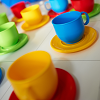#### You may also like### Consecutive Numbers

An investigation involving adding and subtracting sets of consecutive numbers. Lots to find out, lots to explore.### Tea Cups

Place the 16 different combinations of cup/saucer in this 4 by 4 arrangement so that no row or column contains more than one cup or saucer of the same colour.### Chocolate

There are three tables in a room with blocks of chocolate on each. Where would be the best place for each child in the class to sit if they came in one at a time?

# Number Families

##### Age 11 to 14Challenge Level

Children from Beaumaris North Primary School, Melbourne told us:

In our Year 3 Enrichment group we discussed all the possible sets that could be found. Here is what we found!
even numbers {$2, 8, 36, 56, 64, 136$}
odd numbers {$3, 13, 17, 27, 39, 49, 51, 91, 119, 121, 125, 143$}
multiples of one {all of them!}
multiples of $2$ {$2, 8, 36, 56, 64, 136$ - the same as even numbers!!}
multiples of $3$ {$3, 27, 36, 39, 51$}
multiples of $4$ {$8, 36, 56, 64, 136$}
multiples of $7$ {$49, 56, 91, 119$}
multiples of $8$ {$8, 56, 64, 136$}
numbers starting with $1$ {$13, 17, 119, 121, 125, 136, 143$}
square numbers {$36, 49, 64, 121$}
numbers containing $2$ {$2, 27, 121, 125$}
square roots {all of them are square roots !}
numbers containing $1$ {$13, 17, 51, 91, 119, 121, 125, 136, 143$}
numbers containing $6$ {$36, 56, 64, 136$}
cube numbers {$8, 27, 64, 125$}
numbers containing $3$ {$3, 13, 36, 39, 136, 143$}
prime numbers {$2, 3, 13, 17$}
numbers containing $9$ {$39, 49, 91, 119$}
numbers that you can subtract one from {all of them again!}
numbers between one and one hundred and forty four {all of them AGAIN!}
numbers between one and one hundred {$2, 8, 13, 17, 27, 36, 39, 49, 51, 56, 64, 91$}
whole numbers {all of them}
triple digit numbers {$119, 121, 125, 136, 143$}
numbers with double digits {$13, 17, 27, 36, 39, 49, 51, 56, 64, 91$}

We enjoyed this because we liked picking out different possibilities and seeing if they worked out. We could have submitted more but the bell rang! Thanks for the great challenge.

Olly from North Molton Primary also found:

multiples of $13$: {$13, 39, 91, 143$}
multiples of $17$: {$17, 51, 119, 136$}

... and multiples of $1$ which of course is all of the numbers.

Jin and Anushkawho go to Dubai International Academy had one more set:

digits add to $8${$8,17,125,143$}

Can you find any more? How about triangle numbers and tetrahedral numbers?